##### PRODUCTS

You’ve ordered an IMPACK Ergosa packer.

Your new *shiny* Ergosa packer was delivered, installed, and started.

It is now running in full operation and fully supported with a well-trained crew.

You have a great idea of how the Ergosa works, you managed to reduce your packing staff by 1 or 2 people, and you’ve increased your packing speed by 25%, 50%, or even 100%.

“How can I push my Ergosa to its maximum potential?”

“How can I get the greatest return on investment from my Ergosa?”

You start having lots of questions on your mind. Questions like, should I have longer or shorter rows? Should I go for a certain packing configuration over another? Do I need a case sealer? A case erector? What about inspection operations? Where is my biggest "loss" in speed?

You know that the answer to those questions could mean a substantial increase in productivity which means closing more contracts, and hence more cash.

Here’s the truth: There is no easy or direct answer to all those questions.

Given that each company’s production and circumstances are unique and different, maximizing your Ergosa’s productivity is entirely dependent on your company’s specific situation and how you utilize your Ergosa packer.

And because the Ergosa packer has 3 main packing modes, the steps you need to take to maximize your Ergosa’s productivity can vary from one packing mode to the other.

Over the last 10 years, I've worked on many Ergosa packers.

Whether it’s wiring, testing, installing, administering operator training, or recommending them to clients, I’ve seen how the most and least successful box manufacturers utilize their Ergosa packers.

Those experiences prove what actions make box manufacturers realize the greatest and smallest payback from their Ergosa.

This article will outline to you, step-by-step, how you can achieve the maximum possible productivity with the Ergosa’s Horizontal Mode.

I also wrote 2 other articles on how to maximize your productivity with the Ergosa’s Vertical Mode and the Ergosa’s Pick & Place Mode that I’d strongly recommend you to check out if you’re using those packing modes.

## How Much Productivity Can You Get from an Ergosa?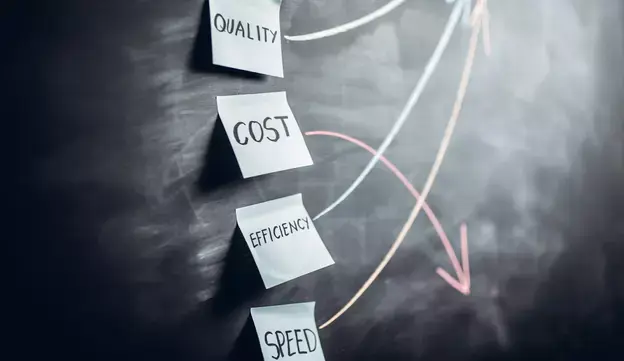Just like with every packing system, the productivity of the Ergosa packer can be defined by a mathematical equation.

This mathematical equation determines how successful you are in maximizing your Ergosa’s productivity regardless of what packing mode you’re using.

### 1. The Mathematical Equation of Packing SpeedThe mathematical equation of the packing speed is simply the formula used to calculate the packing speed.

To define the formula of packing speed under the Ergosa’s Horizontal Mode, I will breakdown the packing process into its 3 main stages:

• Step #1 — Preparation (Pr): The operator prepares the empty case which is to be packed with the boxes.
• Step #2 — Insertion (I): The operator inserts the empty case on the Ergosa’s Case Holding System.
• Step #3 — Packing (P): The operator separates a row of boxes from the shingle and packs them into the case.

Step #3 — Packing (P) also includes all the control operations, labeling, etc. The operator will repeat Step #3 — Packing (P) as many times as there are rows in the case.

For example, if the packing configuration has 4 rows, the operator will repeat Step #3 — Packing (P) 4 times.

Once the row(s) of boxes has been packed into the case, the operator presses the ejection button and the Ergosa will release the case from the Case Holding System. The operator then repeats step #1 to step #3 for each case.

For each of these 3 steps that constitute the packing process, we can define the average time needed for the operator to perform the action.

After many years of repeating this process, constant testing, and regular validations from our clients and experts, here is the predicted average time needed for the operator to perform each step:

• Step #1 — Preparation (Pr)   🡺 7 seconds.
• Step #2 — Insertion (I)           🡺 3 seconds.
• Step #3 — Packing (P)           🡺 15 seconds per row.

Note that this “average time” is rather conservative and does not represent the best productivity you can achieve.

By breaking down the packing process into its 3 main steps, we can define the packing speed with the following formula: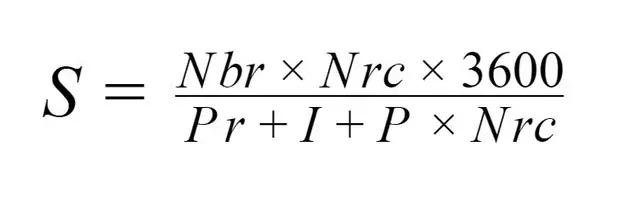Where:

• S = Speed in boxes per hour
• Nbr = Number of boxes per row
• Nrc = Number of rows per case
• 3600 = Number of seconds per hour
• Pr = Preparation time
• I = Insertion time
• P = Packing time per row

For example, if the case consists of 4 rows per case and 400 boxes per row, the packing speed will be:### 2. The Parameters of the Packing Speed’s Equation and How they Influence the Packing Speed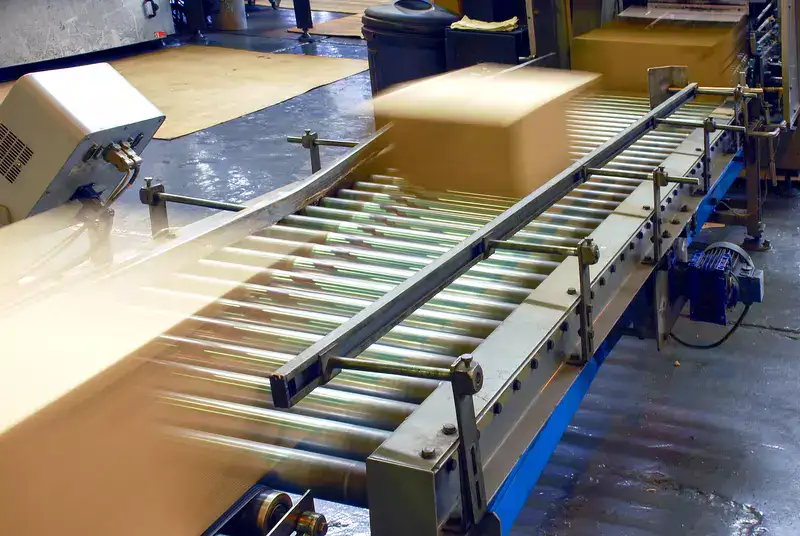Now that we’ve broken down the formula of packing speed and how it is derived, let’s take a closer look at the parameters of this equation and how each parameter affects the packing speed.

We defined the formula of packing speed under the Ergosa’s Horizontal Mode as:For the speed (S) to be as high as possible in value, the numerator (Nbr x Nrc x 3600) must be as high as possible and the denominator (Pr + I + P x Nrc) must be as low as possible.

There are 4 parameters that you can take advantage of to improve this speed (S), and thereby, the productivity of your Ergosa in Horizontal Mode. These 4 parameters are bolded below:

1. S = Speed in boxes per hour
2. Nbr = Number of boxes per row
3. Nrc = Number of rows per case
4. 3600 = Number of seconds per hour
5. Pr = Preparation time
6. I = Insertion time
7. P = Packing time per row

For the other 2 parameters (3600 = Number of seconds per hour and I = Insertion time), you can’t influence them simply because there will always be 3600 seconds in an hour and the insertion time (I) of a case in the Ergosa's Case Holder System cannot be reduced.

Depending on the dexterity and skills of your operator, their level of involvement in the task, and the organization of your workstation, the I = Insertion time may vary slightly but will always be between 2 to 3 seconds.

Alright. Now that we talked about the parameters you cannot influence, let’s divert our attention to what’s important: The 4 parameters you can take advantage of to maximize your Ergosa’s productivity under the Horizontal Mode.

These 4 parameters, again, are 1) Nbr = Number of boxes per row, 2) Nrc = Number of rows per case, 3) Pr = Preparation time, and 4) P = Packing time per row.

### 1. Nbr = Number of boxes per row

The greater the Nbr = Number of boxes per row, the greater your packing speed.

The diagram below shows a visual representation of how increasing the number of boxes per row results in higher packing speeds (Nrc = 1, Pr = 7, I = 3, P = 15).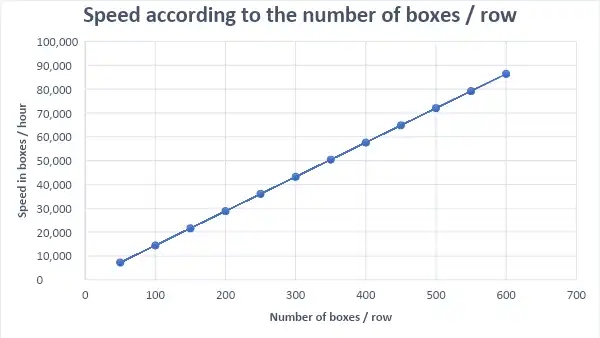“So, if increasing the number of boxes per row increases my packing speed, then should I add as many boxes as I can per row?”

Great question! Increasing your Nbr = Number of boxes per row increases your packing speed up to a point.

In other words, there comes a point where the Nbr = Number of boxes per row are too many for an operator to handle.

“Okay, what’s the ideal number of boxes per row?”

Usually, the ideal number of boxes per row is approximately 400 to 500 boxes per row.

That said, when it comes to defining the number of boxes per row, you have to take into account the weight of the row.

Each row should not exceed 8 to 10 kg. For your Ergosa packer to perform optimally in the long-run with packing staff that can follow, we recommend a maximum weight of 8 to 10 kg per row.

This means that you may have to limit the number of boxes per row to 300 boxes, for example, because of the weight of the row.

Anything above 8 to 10 kg will make life very difficult for your operator/packing person to keep a high packing speed in the long-run.

In sum, to maximize your Ergosa’s productivity, increase your number of boxes per row to the largest amount possible while taking into consideration the weight of the row.

This is usually between 400 to 500 boxes per row.

### 2. Nrc = Number of rows per case

The Nrc = Number of rows per case is the most complex factor to determine since it is present in both the numerator and denominator of the formula of packing speed.

It is, therefore, necessary to find a point of equilibrium given that the Nrc affects the packing speed in both directions.

Let's first examine the Nrc’s presence in the numerator of the packing speed’s equation:###### The formula of packing speed under the Ergosa’s Horizontal Mode.

We said how we want the Nbr = Number of boxes per row to be as large as possible.

We know that the Nbr = Number of boxes per row combined with the Nrc = Number of rows per case together forms the number of boxes per case.

And rationally, we want the number of boxes per case to be as large as possible.

If we now look at the denominator part of the equation, the greater the Nrc = Number of rows per case is, the more it limits the speed gain. While that is true, it acts in conjunction with the Pr = Preparation time and I = Insertion time which have generally "fixed" values.

Hence, it is necessary to find a point of equilibrium between these two phenomena.

The graph below shows how the packing speed changes when the Nbr = Number of boxes per row and the Nrc = Number of rows per case changes.

Here, the Nrc = Number of rows per case increases from 1 row per case to 8 rows per case (Pr = 7, I = 3, P = 15).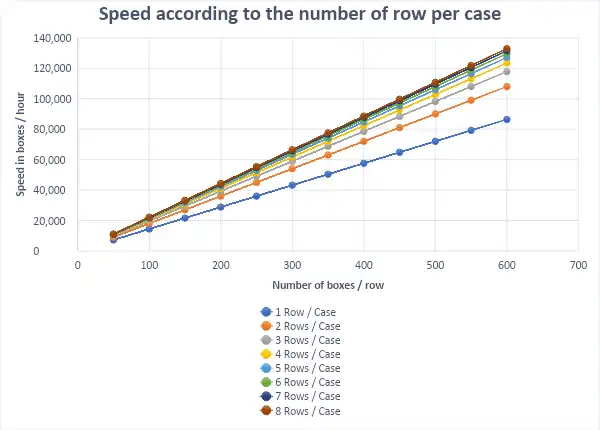As the graph shows, increasing the Nrc = Number of rows per case increases the packing speed but up to a point.

That is, there comes a point where increasing the Nrc = Number of rows per case results in a smaller increase in the packing speed.

More specifically, we can see that increasing the Nrc = Number of rows per case from 1 to 4 rows increases your packing speed by approximately 40,000 boxes per hour.

In contrast, increasing the Nrc = Number of rows per case from 4 to 8 rows increases your packing speed by only 10,000 boxes per hour i.e. 4 times less.

“What’s the ideal number of rows per case?”

The answer is as large as possible. Without diving into too many mathematical details, each additional row increases your packing speed.

However, after you reach a certain number of rows, the gain in your packing speed becomes very limited. And when you are handling full cases, you’ll face limitations due to the weight of the case.

Clients that perform at high speeds with the Ergosa’s Horizontal Mode typically have 3 or 4 rows per case for standard sized boxes. For small boxes, such as pharmaceutical boxes, they usually have 6 to 8 rows per case.

In sum, to maximize your Ergosa’s productivity, increase your number of rows per case to the largest amount possible while taking into consideration the weight of the case.

This is usually between 3 to 4 rows per case for standard sized boxes and 6 to 8 rows per case for small sized boxes.

### 3. Pr = Preparation time

The Pr = Preparation time is the time it takes an operator to prepare each empty case so that it can be packed with the boxes.

The Pr’s value is either 0 or 7 seconds.

If the operator in charge of packing boxes into cases is also responsible for preparing empty cases, then the Pr’s value is 7 seconds.

If your packing station is equipped with a case erector or you have a separate operator in charge of preparing empty cases, then the Pr’s value is 0 seconds.

Having a case erector influences the impact the Nbr = Number of boxes per row has on the speed (S).

To better understand how a case erector influences your speed (S), we put 2 examples that shows what happens to the speed (S) when the Nbr = Number of boxes per row is increased if you do not have a case erector versus if you do have a case erector.

With just 1 row per case, it is obvious that having a case erector increases the speed considerably versus when not having a case erector.

In fact, the gain in packing speed with a case erector is about ±39% higher than without a case erector.

Let’s take an example of 2 situations with having and without having a case erector (Nbr = 500, Nrc = 1, I = 3 & P = 15).

• With a case erector (Pr = 0) the speed (S) will be: 100,000 boxes per hour.
• Without a case erector (Pr = 7) the speed (S) will be: 72,000 boxes per hour.

This represents approximately a 39% packing speed difference.

However, the more rows you have, the less the gain in speed with a case erector (Nbr = 500, Nrc = 4, I = 3 & P = 15).

• With a case erector (Pr = 0) the speed (S) will be : 114,286 boxes per hour.
• Without a case erector (Pr = 7) the speed (S) will be : 102,857 boxes per hour.

This represents approximately an 11% packing speed difference.

### 4. P = Packing time per row

The P = Packing time per row refers to the time needed to pack each row of boxes into the case.

The P = Packing time per row depends on two things: First, the packing person’s (or operator’s) level of involvement in the packing, and second, the number of operations this packing person/operator has to perform.

If the packing person has to also carry out controls, label the boxes, take samples for quality control, etc. in addition to packing rows into cases, then the P = Packing time per row can vary from 10 to 15 seconds (but we’ve seen well organized company operators in this same situation capable of performing the operation in 7 seconds only).

In-line automatic quality control systems, such as those recommended for the glue control unit, will allow you to automate these quality-control checks and thus reduce the number of actions that the packing person/operator will have to perform.

Finally, the organization of your packing station plays a fundamental role.

If everything is adapted such that it makes the workload simple and consistent for your packing staff, then your packing person/operator(s) will be able to reduce their P = Packing time.

For this reason, we recommend 10 mm to 15 mm (single or multi-row) of clearance between the width of the box and the case.

Furthermore, we recommend 50 mm clearance when the row is slightly compressed to allow a smooth and hassle-free packing process.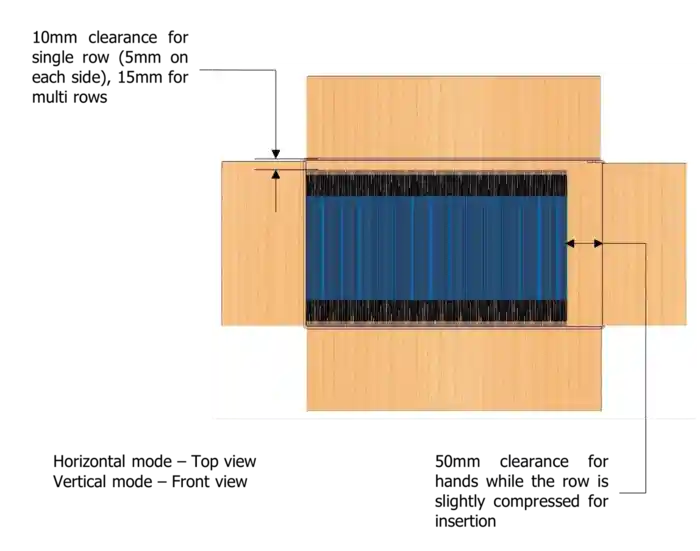The following graph shows the gain in packing speed between a P = Packing time of 10 seconds versus 12 seconds versus 15 seconds.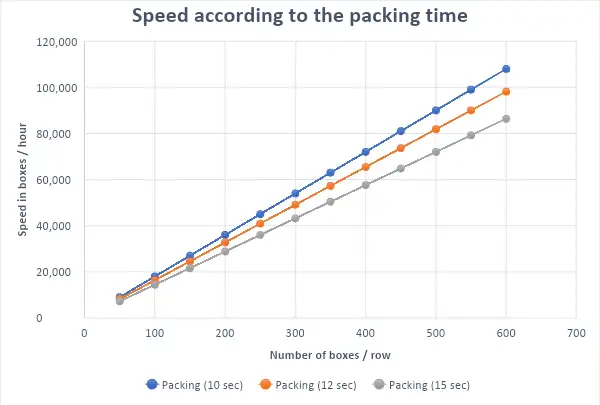As shown in the graph, a better organization of the workstation combined with a well organized operator that does not have to do tasks outside of the packing can result in a reduction in the packing time (P) from 15 seconds to 12 seconds and even down to 10 seconds.

You should also know that the boxes themselves can influence the P = Packing time.

The box’s shape, size, type, paint, or varnish, can influence the difficulty of packing each row into the case, and thus, the P = Packing time per row. This, thereby, influences the packing speed (S).

## How to Get the Greatest Return on Investment From Your Ergosa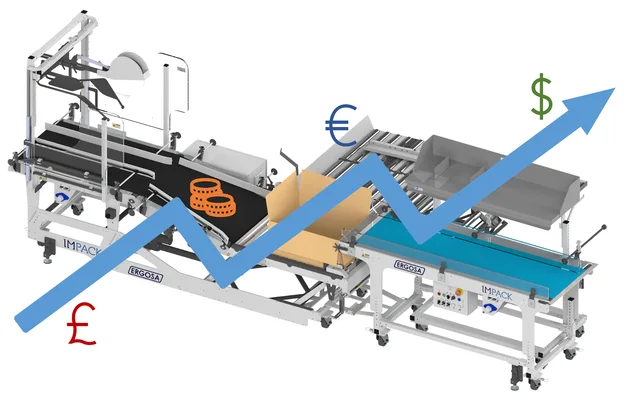By now, you should have a solid understanding of how to maximize your Ergosa’s productivity under the Horizontal Mode

But, to get the greatest return on investment from your Ergosa packer, you’d want to maximize your Ergosa’s productivity under every packing mode you are using.

How do you do that? We’ve got you covered!

Check out the following two guides that show you exactly how to maximize your Ergosa’s productivity based on the packing mode(s) your company is using:

Got more questions that you want answers for?

Contact IMPACK’s Experts for help! They can answer all your questions and determine the best solutions for the problems you’re experiencing, commitment-free.